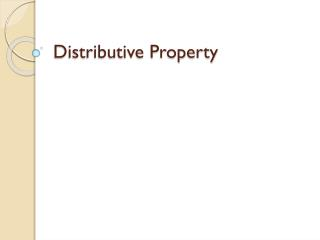DownloadDownload PresentationDistributive Property

# Distributive Property

Télécharger la présentation## Distributive Property

- - - - - - - - - - - - - - - - - - - - - - - - - - - E N D - - - - - - - - - - - - - - - - - - - - - - - - - - -
##### Presentation Transcript

1. Distributive Property

2. The Distributive Property-When two addends are multiplied by a factor, the product is the same as if each addend was by the factor and those products were addedExample: a x (b+c) = (a x b) + (a x c) or 4 x 13 = 4 x (10 + 3) = (4 x 10) + (4 x 3)

3. This is how we distribute • 5 x (20 + 3) • 5 x 20 = 5 x 3 = • 100 + 15 = 115 • Try the four on your notes

4. The distributive property is mental math strategy that can be used when multiplying. 43 x 5 =?

5. Break apart the double-digit number. 43 x 5 =? 40 3 +

6. Then multiply each part by 5. 43 x 5 =? 40 3 + x 5x 5

7. Then multiply each part by 5. 43 x 5 =? 40 3 + x 5x 5 200 15

8. Finally, sum your two products 43 x 5 =215 40 3 + x 5x 5 200 15 + = 215

9. Let’s look at another example. 53 x 6 = ?

10. Break apart the double-digit number. 53 x 6 = ?

11. Break apart the double-digit number. 53 x 6 = ? 50 3 +

12. Multiply each part by 6. 53 x 6 = ? 50 3 + x 6x 6

13. Multiply each part by 6. 53 x 6 = ? 50 3 + x 6x 6 300 18

14. Sum the two products. 53 x 6 = 318 50 3 + x 6x 6 300 + 18 = 318

15. There are three steps to the distributive property. 4 x 28 = 1) Break apart the double-digit number.

16. There are three steps to the distributive property. 4 x 28 = 4 x (20 + 8) 1) Break apart the double-digit number.

17. There are three steps to the distributive property. 4 x 28 = 4 x (20 + 8) • Break apart the double-digit number. • Multiply each part by 4. (distribute the 4)

18. There are three steps to the distributive property. 4 x 28 = 4 x (20 + 8) = (4 x 20) + (4 x 8) • Break apart the double-digit number. • Multiply each part by 4. (distribute the 4)

19. There are three steps to the distributive property. 4 x 28 = 4 x (20 + 8) = (4 x 20) + (4 x 8) • Break apart the double-digit number. • Multiply each part by 4. (distribute the 4) • Sum the two products.

20. There are three steps to the distributive property. 4 x 28 = 4 x (20 + 8) = 80 + (4 x 8) • Break apart the double-digit number. • Multiply each part by 4. (distribute the 4) • Sum the two products.

21. There are three steps to the distributive property. 4 x 28 = 4 x (20 + 8) = 80 + 32 • Break apart the double-digit number. • Multiply each part by 4. (distribute the 4) • Sum the two products.

22. There are three steps to the distributive property. 4 x 28 = 4 x (20 + 8) = 80 + 32 = 112 • Break apart the double-digit number. • Multiply each part by 4. (distribute the 4) • Sum the two products.

23. In this example, the 5 was distributed. 5 x 38 = 5 x (30 + 8) = (5 x 30) + (5 x 8)

24. In this example, the 7 was distributed. 7 x 46 = 7 x (40 + 6) = (7 x 40) + (7 x 6)

25. Practice Time

26. 1) Which of the following expressions shows the distributive property for 5 x (3 + 7)? (5 + 3) x (5 + 7) (5 x 3) x (5 x 7) (5 x 3) + (5 x 7)

27. 1) Which of the following expressions shows the distributive property for 5 x (3 + 7)? Correct! (5 x 3) + (5 x 7)

28. 2) Which of the following expressions shows the distributive property for 3 x (9 + 4) ? (3 x 9) + (3 x 4) (3 + 9) + (3 + 4) (3 + 9) x (3 + 4)

29. 2) Which of the following expressions shows the distributive property for 3 x (9 + 4) ? (3 x 9) + (3 x 4) Correct!

30. 5) Which of the following expressions is equivalent to:(5 x 9) + (5 x 3) ? 3 x (9 + 5) 5 x (9 + 3) 9 x (3 + 5)